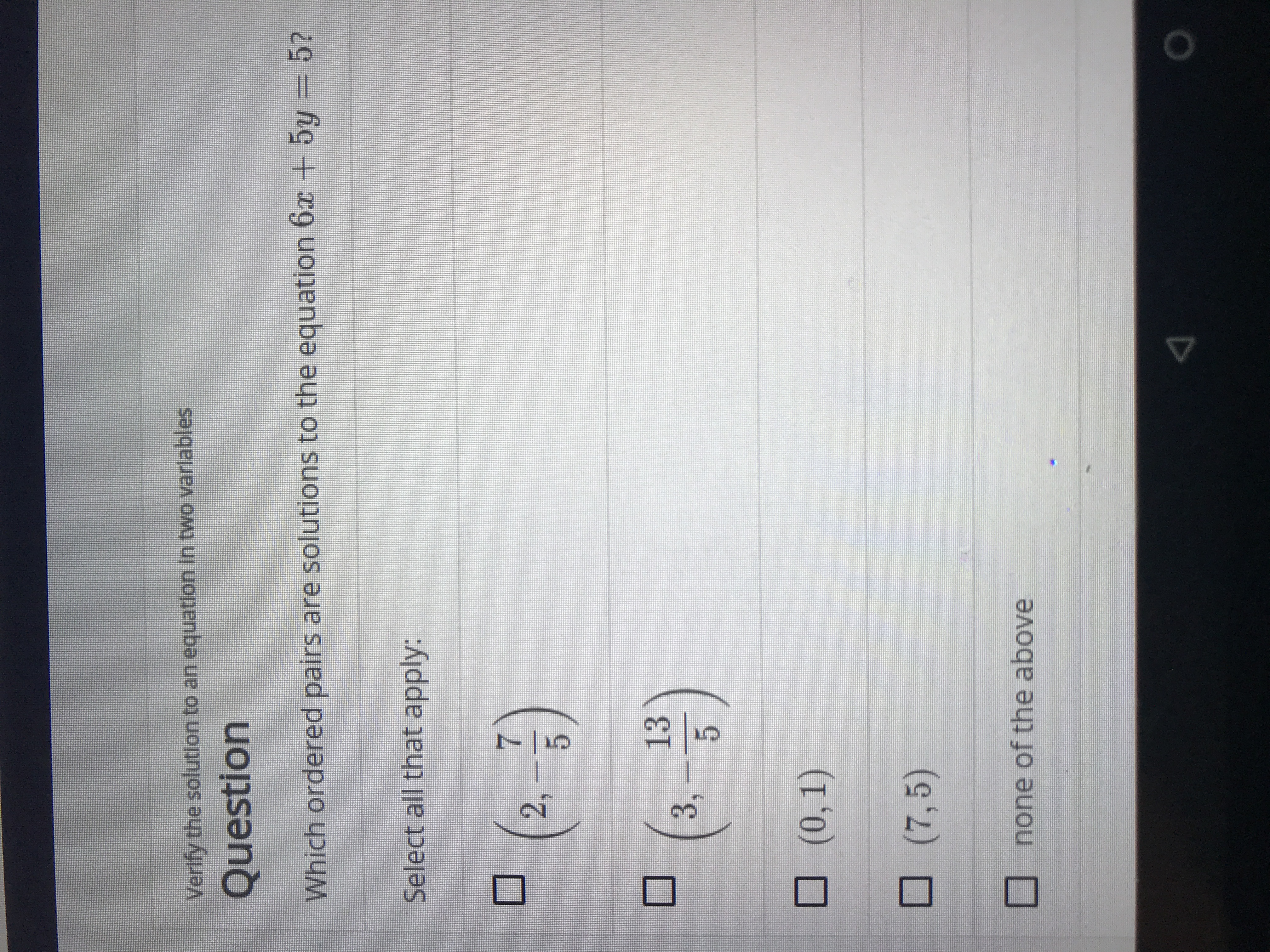# Verlfy the solution to an equatlon In two varlablesQuestionWhich ordered pairs are solutions to the equation 6x + 5y = 5?Select all that apply:2,133,(0, 1)(7,5)none of the above

Questionhelp_outlineImage TranscriptioncloseVerlfy the solution to an equatlon In two varlables Question Which ordered pairs are solutions to the equation 6x + 5y = 5? Select all that apply: 2, 13 3, (0, 1) (7,5) none of the above fullscreen
check_circleExpert Solution
Step 1

We plug the given points and check.

(2, -7/5) , so x=2 and y=-7/5

This works.

Step 2

(3, -13/5) , so x=3 and y=-13/5

This works.

Step 3

(0, 1) , so x=0 and y=1

...

### Want to see the full answer?

See Solution

#### Want to see this answer and more?

Solutions are written by subject experts who are available 24/7. Questions are typically answered within 1 hour*

See Solution
*Response times may vary by subject and question
Tagged in

### Other